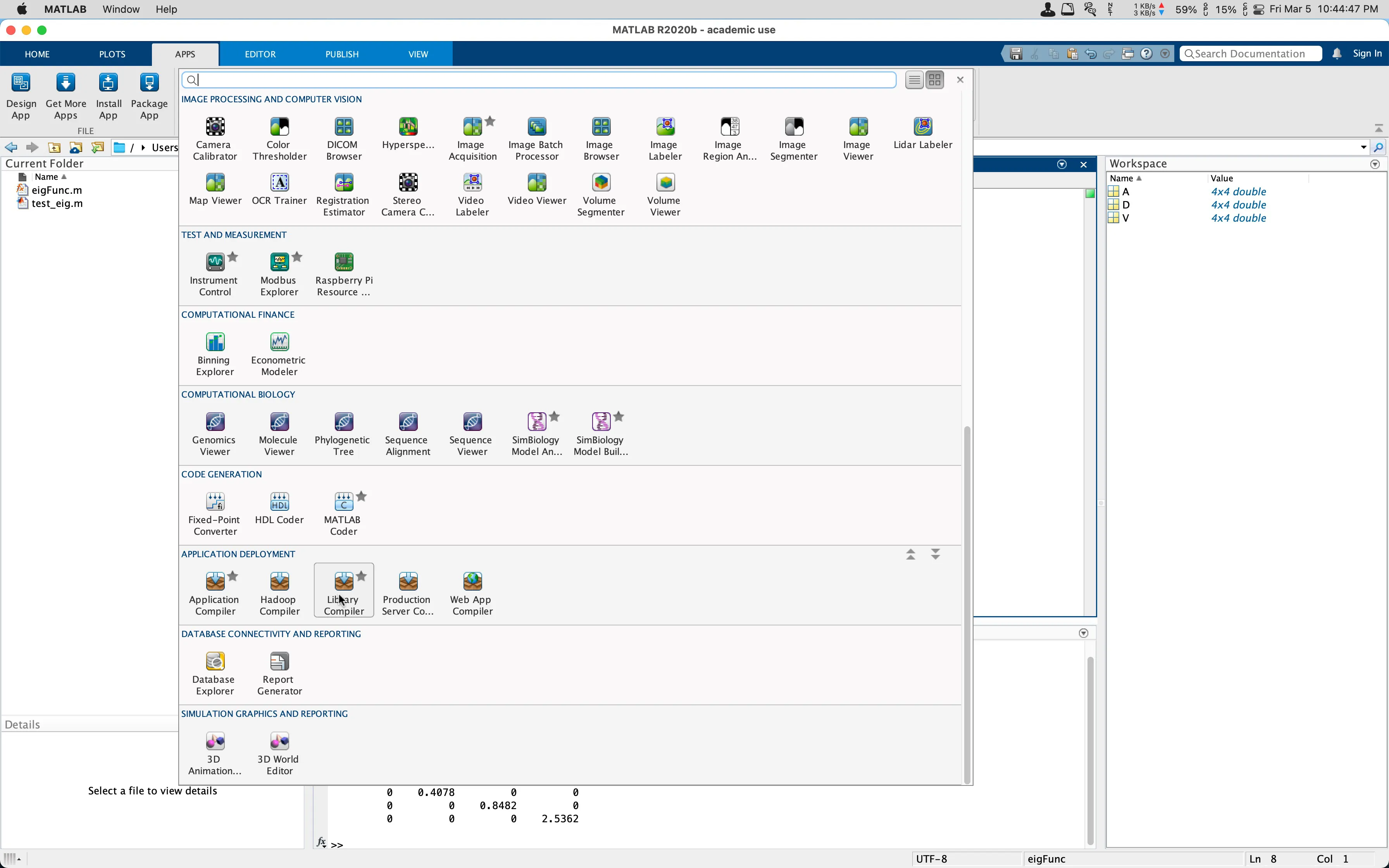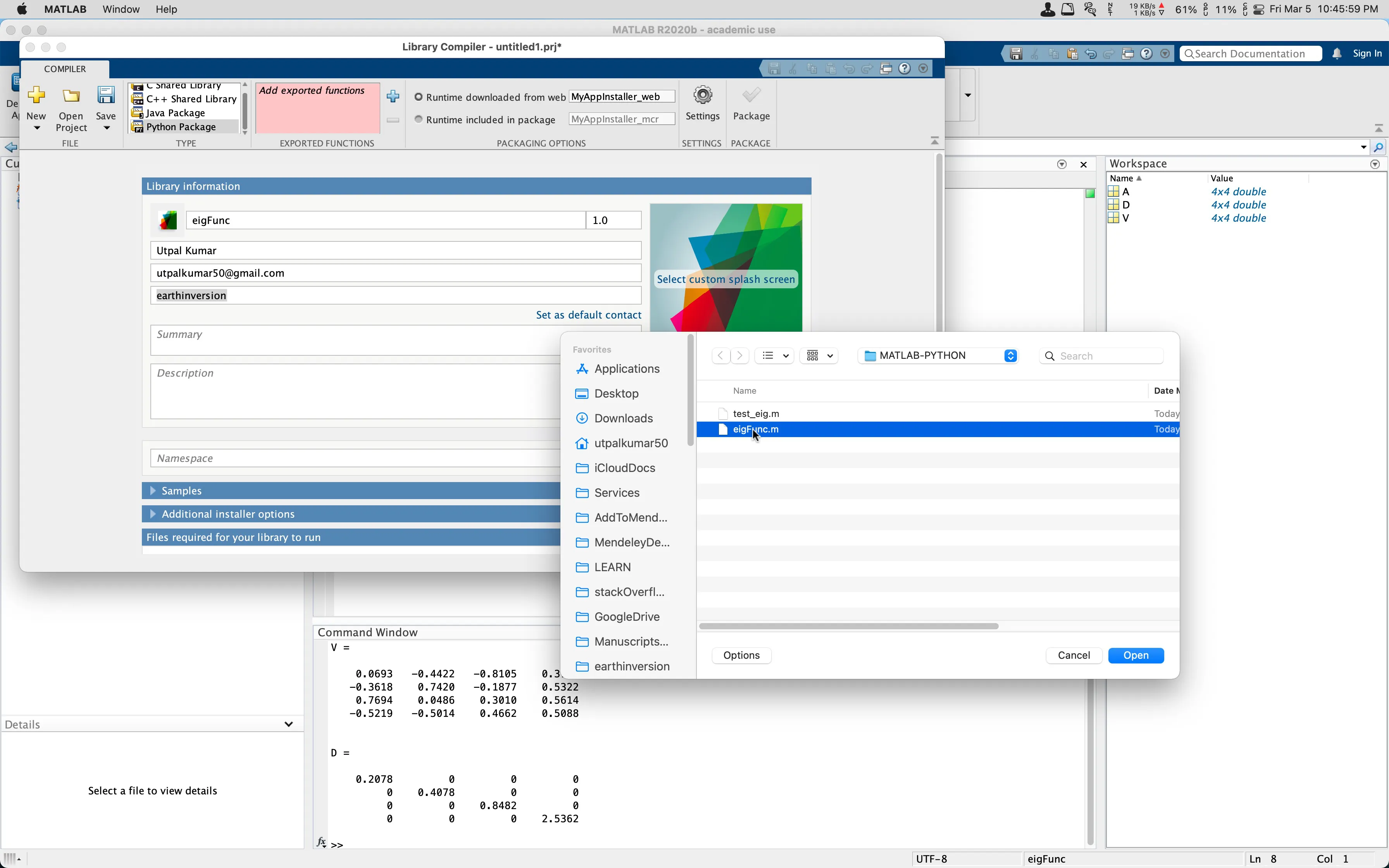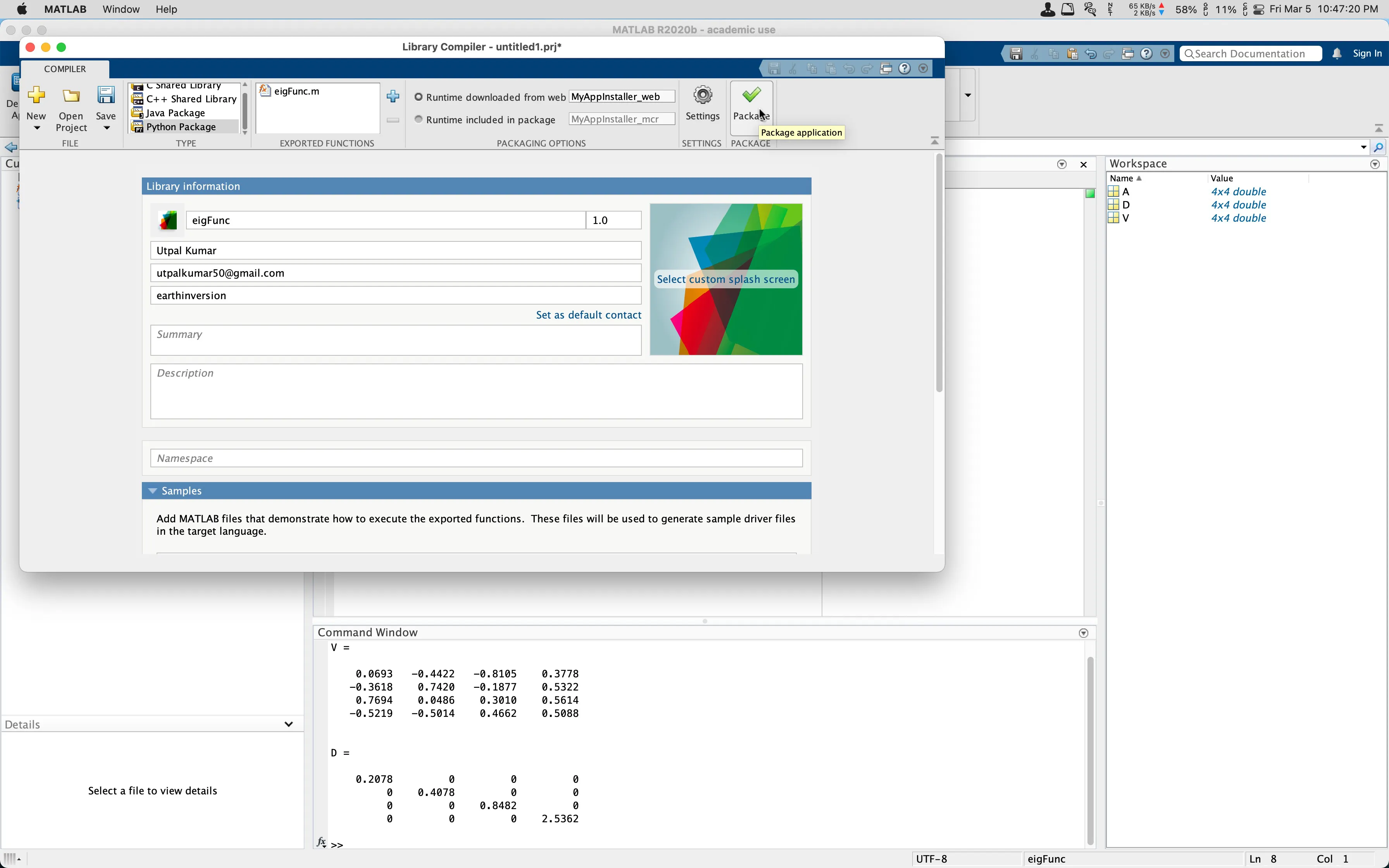# Easily integrate Custom Functions in MATLAB with Python (codes included)

How can we use the MATLAB functions in Python? MATLAB implementation are usually reliable as it is developed by the professionals. But the advantages of using Python for scripting is immense.

## Introduction

MATLAB implementation are usually quite reliable as it is developed by the professionals. But the advantages of using Python is immense. In this post, I will show how you can benefit the MATLAB function in your Python script.

## Defining custom function in MATLAB

Let us make a custom function in MATLAB that we can use in Python. For the purpose of demonstration, I will use a very simple function but the same idea applies to any function.

## Similar posts

### Eigenvalues and eigenvectors in MATLAB

function [V,D] = eigFunc(A)
%returns diagonal matrix D of eigenvalues and matrix V
% whose columns are the corresponding right eigenvectors,
% so that A*V = V*D.
[V, D] = eig(A);
end


I saved the above function as eigFunc. This function takes in a square matrix as input and outputs diagonal matrix D of eigenvalues and matrix V whose columns are the corresponding right eigenvectors [see eig function in MATLAB].

Let’s first use this function in MATLAB for test purpose.

clear; close all; clc
A = gallery('lehmer',4);

[V,D] = eigFunc(A)



This returns:

V =

0.0693   -0.4422   -0.8105    0.3778
-0.3618    0.7420   -0.1877    0.5322
0.7694    0.0486    0.3010    0.5614
-0.5219   -0.5014    0.4662    0.5088

D =

0.2078         0         0         0
0    0.4078         0         0
0         0    0.8482         0
0         0         0    2.5362


This works great in MATLAB as expected (coz the function is exact copy of the eig function in MATLAB. But how can we use this function in Python?

## Time-Frequency Analysis in MATLAB

A signal has one or more frequency components in it and can be viewed from two different standpoints: time-domain and frequency domain. In general, signals a...

## Call MATLAB in Python

### Using MATLAB Engine API for Python

The easiest way to use the matlab function in Python is by using the matlab.engine. You can install matlab library by following these two ways.

#### Install from inside MATLAB:

cd (fullfile(matlabroot,'extern','engines','python'))
system('python setup.py install')


#### Install directly from terminal:

Navigate to the MATLAB source location and compile the python. For installing matlab library in Mac:

cd /Applications/MATLAB_R2021b.app/extern/engines/python/
python setup.py install


Please note that this MATLAB engine API will be installed for the the specific version of python. If you are using anaconda, you can inspect the version of Python you are installing the MATLAB engine API for. It is essentially the same way you install other Python libraries. For details, visit here.

import matlab
import matlab.engine
eng = matlab.engine.start_matlab()
A = [[1.0000,    0.5000,    0.3333,    0.2500],
[0.5000,    1.0000,    0.6667,    0.5000],
[0.3333,    0.6667,    1.0000,    0.7500],
[0.2500,    0.5000,    0.7500,    1.0000]]
A = matlab.double(A)
V, D = eng.eigFunc(A, nargout=2)
print("V: ", V)
print("D: ", D)

eng.quit()


Here is the output:

V:  [[0.06939950784450351,-0.4421928183150595,-0.8104910184495989,0.37782737957175255],				[-0.3619020163563876,0.7419860358173743,-0.18770341448628555,0.5322133795757004],[0.7693553355549393,0.04873539080548356,0.30097912769034274,0.5613633351323756],[-0.5218266058004974,-0.5015447096377744,0.4661365700065611,0.5087893432606572]]

D:  [[0.20775336892808516,0.0,0.0,0.0],[0.0,0.40783672775946245,0.0,0.0],[0.0,0.0,0.8482416513967358,0.0],[0.0,0.0,0.0,2.536168251915717]]


The results are same as before. The nargout argument tells the matlab based function to output 2 results here.

## Test your scientific hypothesis in MATLAB

Numerical validation of hypothesis: Is the mean of one population significantly different than the other?

## Create Python Package: Using MATLAB Compiler & MATLAB Runtime

This is all well and good if you have MATLAB installed in your system. But what if you wanna give your Python script so someone who does not have MATLAB installed on their system. In that case, you can build a Python library using the library compiler app in MATLAB. For details, visit Generate a Python Package and Build a Python Application.(a) Select library compiler app in MATLAB (b) Fill in information regarding the Python library(a) Select MATLAB function to generate the Python library (b) Add example MATLAB script(a) Click on package to generate standalone component (b) Wait for the task to finish

#### MATLAB Runtime installation

Please note that, the user need to install MATLAB runtime for successfully using this library. MATLAB runtime helps in running compiled MATLAB applications or components without installing MATLAB. The runtime can be downloaded from here for Windows, Mac and Linux OS and it is free to download.

#### Import MATLAB based library for Python

import eigFunc
eigFuncAnalyzer = eigFunc.initialize() #calls the matlab runtime
A = [[1.0000,    0.5000,    0.3333,    0.2500],
[0.5000,    1.0000,    0.6667,    0.5000],
[0.3333,    0.6667,    1.0000,    0.7500],
[0.2500,    0.5000,    0.7500,    1.0000]]
A = array.array(A) %not tested
V, D = eigFuncAnalyzer.eigFunc(A, nargout=2)
print("V: ", V)
print("D: ", D)
eigFuncAnalyzer.terminate()


Please note that you can design your eigFunc.m in a way that you can simply load the mat data and you can use scipy.io.savemat function to save the python data to mat format. For details see the scipy documentation.

## Data Type Conversions

MATLAB PYTHON
double, single float
complex single complex double complex
(u)int8, (u)int16, (u)int32,(u)int64 int
NaN float(nan)
Inf float(inf)
String, char str
Logical bool
Structure dict
Vectors array.array()
Cell array list, tuple